# Calculating week number in Excel (WEEKNUM function)

While Microsoft Excel provides an array of functions to work with weekdays, months and years, only one is available for weeks - the WEEKNUM function. So, if you are looking for a way to get a week number from a date, WEEKNUM is the function you want.

In this short tutorial, we will briefly talk about the syntax and arguments of Excel WEEKNUM, and then discuss a few formula examples demonstrating how you can use the WEEKNUM function to calculate week numbers in your Excel worksheets.

## Excel WEEKNUM function - syntax

The WEEKNUM function is used in Excel to return the week number of a specific date in the year (a number between 1 and 54). It has two arguments, the 1st is required and the 2nd is optional:

WEEKNUM(serial_number, [return_type])
• Serial_number - any date within the week whose number you are trying to find. This can be a reference to a cell containing the date, a date entered by using the DATE function or returned by some other formula.
• Return_type (optional) - a number that determines on which day the week begins. If omitted, the default type 1 is used (the week beginning on Sunday).

Here is a complete list of the `return_type` values supported in WEEKNUM formulas.

 Return_type Week begins on 1 or 17 or omitted Sunday 2 or 11 Monday 12 Tuesday 13 Wednesday 14 Thursday 15 Friday 16 Saturday 21 Monday (used in System 2, please see the details below.)

In the WEEKNUM function, two different week numbering systems are used:

• System 1. The week containing January 1 is considered the 1st week of the year and is numbered week 1. In this system, the week traditionally starts on Sunday.
• System 2. This is the ISO week date system that is part of the ISO 8601 date and time standard. In this system, the week starts on Monday and the week containing the first Thursday of the year is considered week 1. It is commonly known as the European week numbering system and it is used mainly in government and business for fiscal years and timekeeping.

All of the return types listed above apply to System 1, except for return type 21 that is used in System 2.

Note. In Excel 2007 and earlier versions, only options 1 and 2 are available. Return types 11 through 21 are supported in Excel 2010 and Excel 2013 only.

## Excel WEEKNUM formulas to convert date to week number (from 1 to 54)

The following screenshot demonstrates how you can get week numbers from dates with the simplest `=WEEKNUM(A2)` formula: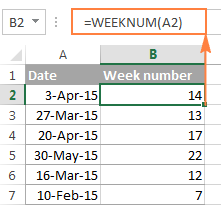In the above formula, the `return_type` argument is omitted, which means that the default type 1 is used - the week beginning on Sunday.

If you'd rather begin with some other day of the week, say Monday, then use 2 in the second argument:

`=WEEKNUM(A2, 2)`

Instead of referring to a cell, you can specify the date directly in the formula by using the DATE(year, month, day) function, for example:

`=WEEKNUM(DATE(2015,4,15), 2)`

The above formula returns 16, which is the number of the week containing April 15, 2015, with a week beginning on Monday.

In real-life scenarios, the Excel WEEKNUM function is rarely used on its own. Most often you would use it in combination with other functions to perform various calculations based on the week number, as demonstrated in further examples.

## How to convert week number to date in Excel

As you have just seen, it's no big deal to turn a date into a week number using the Excel WEEKNUM function. But what if you are looking for the opposite, i.e. converting a week number to a date? Alas, there is no Excel function that could do this straight away. So, we will have to construct our own formulas.

Supposing you have a year in cell A2 and a week number in B2, and now you want to calculate the Start and End dates in this week.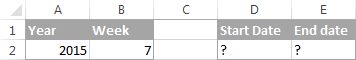Note. This formula example is based on ISO week numbers, with a week starting on Monday.

The formula to return the Start date of the week is as follows:

`=DATE(A2, 1, -2) - WEEKDAY(DATE(A2, 1, 3)) + B2 * 7`

Where A2 is the year and B2 is the week number.

Please note that the formula returns the date as a serial number, and to have it displayed as a date, you need to format the cell accordingly. You can find the detailed instructions in Changing date format in Excel. And here is the result returned by the formula: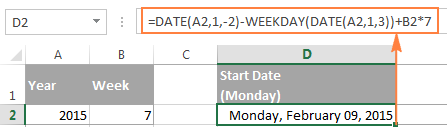Of course, the formula to convert a week number to a date is not trivial, and it may take a while to get your head round the logic. Anyway, I will do my best to provide meaningful explanation for those who are curious to get down to the bottom.

As you see, our formula consists of 2 parts:

• `DATE(A2, 1, -2) - WEEKDAY(DATE(A2, 1, 3))` - calculates the date of the last Monday in the previous year.
• `B2 * 7` - adds the number of weeks multiplied by 7 (the number of days in a week) to get the Monday (start date) of the week in question.

In the ISO week numbering system, week 1 is the week containing the first Thursday of the year. Consequently, the first Monday is always between December 29 and January 4. So, to find that date, we have to find the Monday immediately before January 5.

In Microsoft Excel, you can extract a day of week from a date by using the WEEKDAY function. And you can use the following generic formula to get Monday immediately before any given date:

=date - WEEKDAY(date - 2)

If our ultimate goal were to find Monday immediately before the 5th of January of the year in A2, we could use the following DATE functions:

`=DATE(A2,1,5) - WEEKDAY(DATE(A2,1,3))`

But what we actually need is not the first Monday of this year, but rather the last Monday of the previous year. So, you have to subtract 7 days from January 5 and hence you get -2 in the first DATE function:

`=DATE(A2,1,-2) - WEEKDAY(DATE(A2,1,3))`

Compared to the tricky formula you have just learned, calculating the End date of the week is a piece of cake :) To get Sunday of the week in question, you simply add 6 days to the Start date, i.e. `=D2+6`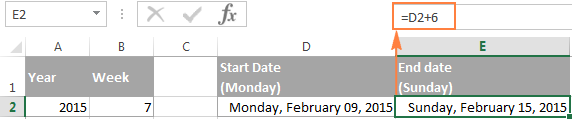Alternatively, you could add 6 directly in the formula:

`=DATE(A2, 1, -2) - WEEKDAY(DATE(A2, 1, 3)) + B2 * 7 + 6`

To make sure the formulas always deliver the right dates, please have a look at the following screenshot. The Start Date and End Date formulas discussed above are copied across column D and E, respectively: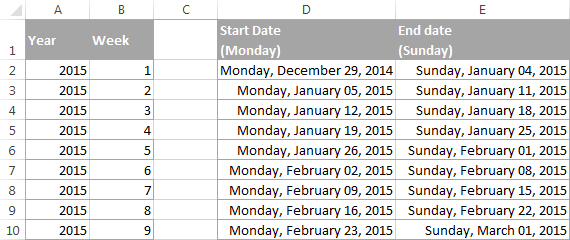### Other ways to convert week number to date in Excel

If the above formula based on the ISO week date system does not meet your requirements, try one of the following solutions.

#### Formula 1. A week containing Jan-1 is week 1, Mon-Sun week

As you remember, the previous formula works based on the ISO date system where the first Thursday of the year is considered week 1. If you work based on a date system where the week containing the 1st of January is considered week 1, use the following formulas:

Start date:

`=DATE(A2,1,1) - WEEKDAY(DATE(A2,1,1),2) + (B2-1)*7 + 1`

End date:

`=DATE(A2,1,1)- WEEKDAY(DATE(A2,1,1),2) + B2*7`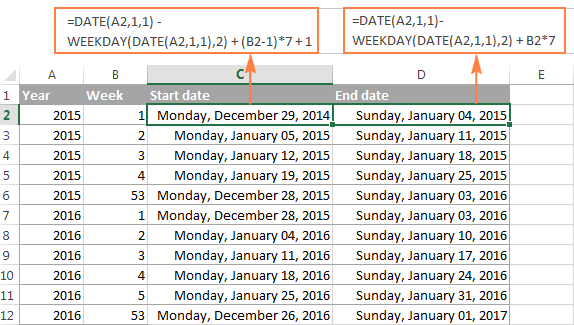#### Formula 2. A week containing Jan-1 is week 1, Sun-Sat week

These formulas are similar to the above ones with the only difference that they are written for Sunday - Saturday week.

Start date:
`=DATE(A2,1,1) - WEEKDAY(DATE(A2,1,1),1) + (B2-1)*7 + 1`

End date:
`=DATE(A2,1,1)- WEEKDAY(DATE(A2,1,1),1) + B2*7`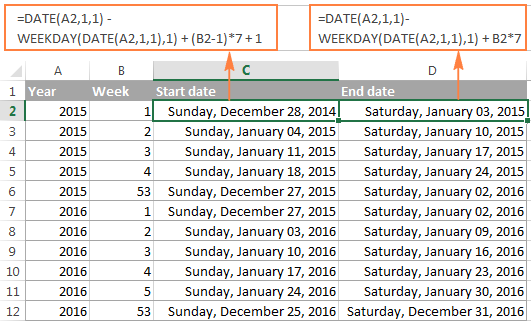#### Formula 3. Always start counting on January 1, Mon-Sun week

While the previous formulas return Monday (or Sunday) of week 1, regardless of whether if falls within this year or the previous year, this start date formula always returns January 1 as the start date of week 1 regardless of the day of the week. By analogy, the end date formula always returns December 31 as the end date of the last week in the year, regardless of the day of the week. In all other respects, these formulas work similarly to Formula 1 above.

Start date:
`=MAX(DATE(A2,1,1), DATE(A2,1,1) - WEEKDAY(DATE(A2,1,1),2) + (B2-1)*7 + 1)`

End date:
`=MIN(DATE(A2+1,1,0), DATE(A2,1,1) - WEEKDAY(DATE(A2,1,1),2) + B2*7)`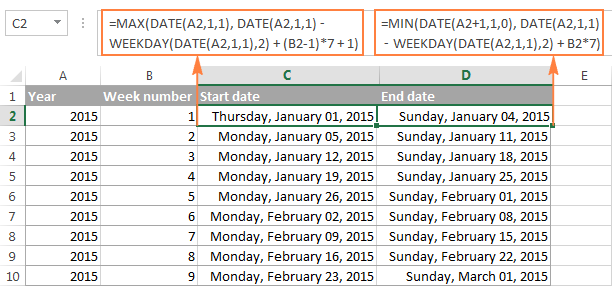#### Formula 4. Always start counting on January 1, Sun-Sat week

To calculate the start and end dates for a Sunday - Saturday week, all it takes is one small adjustment in the above formulas :)

Start date:
`=MAX(DATE(A2,1,1), DATE(A2,1,1) - WEEKDAY(DATE(A2,1,1),1) + (B2-1)*7 + 1)`

End date:
`=MIN(DATE(A2+1,1,0), DATE(A2,1,1) - WEEKDAY(DATE(A2,1,1),1) + B2*7)`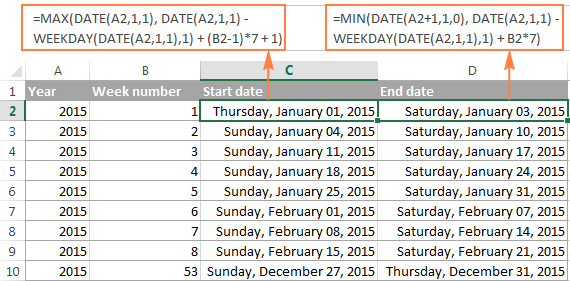## How to get month from week number

To get a month corresponding to the week number, you find the first day in a given week as explained in this example, and then wrap that formula in the Excel MONTH function like this:

`=MONTH(DATE(A2, 1, -2) - WEEKDAY(DATE(A2, 1, 3)) + B2 * 7)`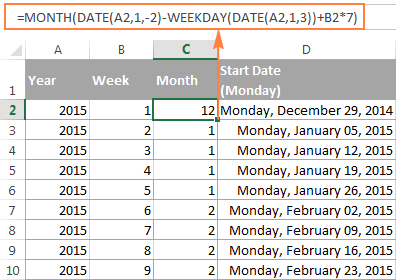Note. Please remember that the above formula works based on the ISO week date system, where the week starts on Monday and the week containing the 1st Thursday of the year is considered week 1. For example, in the year 2016, the first Thursday is January 7, and that is why week 1 begins on 4-Jan-2016.

## How to get a week number in a month (from 1 to 6)

If your business logic requires converting a specific date to the week number within the corresponding month, you can use the combination of WEEKNUM, DATE and MONTH functions:

Assuming that cell A2 contains the original date, use the following formula for a week beginning on Monday (notice 21 in WEEKNUM's return_type argument):

`=WEEKNUM(\$A2,21)-WEEKNUM(DATE(YEAR(\$A2), MONTH(\$A2),1),21)+1`

For a week beginning on Sunday, omit the return_type argument:

`=WEEKNUM(\$A2)-WEEKNUM(DATE(YEAR(\$A2), MONTH(\$A2),1))+1`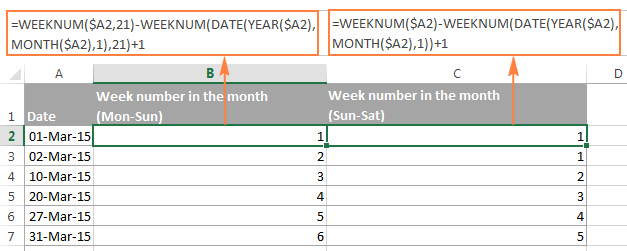## How to sum values and find average by the week number

Now that you know how to convert a date to a week number in Excel, let's see how you can use week numbers in other calculations.

Suppose, you have some monthly sales figures and you want to know the total for each week.

To begin with, let's find out a week number corresponding to each sale. If your dates are in column A and sales in column B, copy the `=WEEKNUM(A2)` formula across column C beginning in cell C2.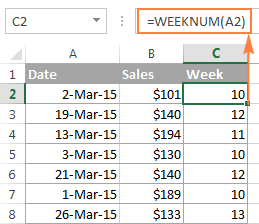And then, make a list of week numbers in some other column (say, in column E) and calculate the sales for each week using the following SUMIF formula:

`=SUMIF(\$C\$2:\$C\$15, \$E2, \$B\$2:\$B\$15)`

Where E2 is the week number.

In this example, we are working with a list of March sales, so we have week numbers 10 to 14, as demonstrated in the following screenshot: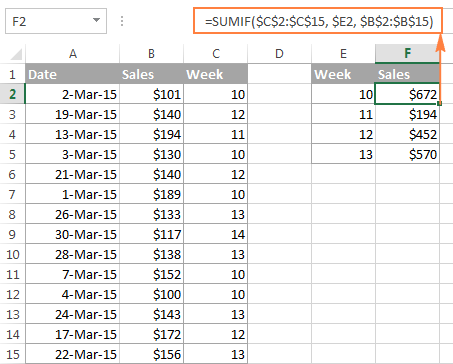In a similar manner, you can calculate the sales average for a given week:

`=AVERAGEIF(\$C\$2:\$C\$15, \$E2, \$B\$2:\$B\$15)`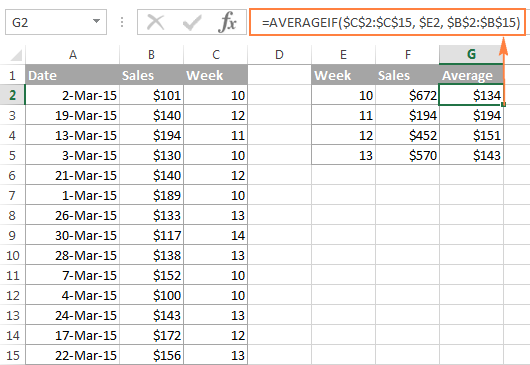If the helper column with the WEEKNUM formula does not fit well into your data layout, I regret to tell you that there is no simple way to get rid of it because Excel WEEKNUM is one of those functions that doesn't accept range arguments. Therefore, it cannot be used within SUMPRODUCT or any other array formula like the MONTH function in a similar scenario.

## How to highlight cells based on the week number

Let's say you have a long list of dates in some column and you want to highlight only those that relate to a given week. All you need is a conditional formatting rule with a WEEKNUM formula similar to this:

`=WEEKNUM(\$A2)=10`

As demonstrated in the screenshot below, the rule highlights sales that were made within week 10, which is the first week in March 2015. Since the rule applies to A2:B15, it highlights values in both columns. You can learn more about creating conditional formatting rules in this tutorial: Excel conditional formatting based on another cell value.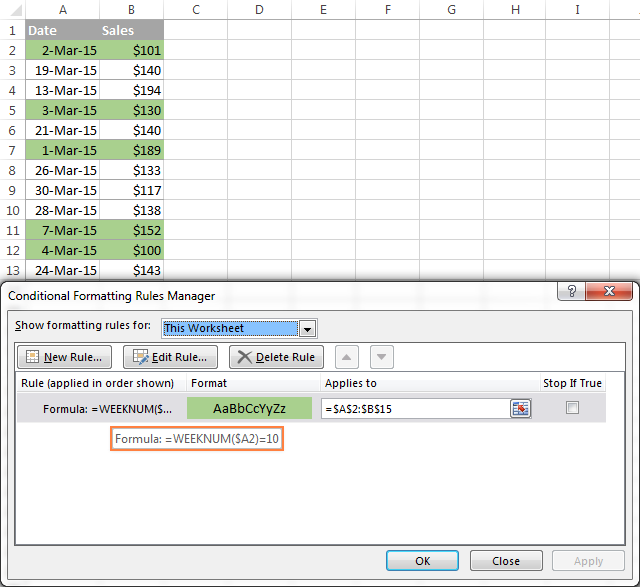This is how you can calculate week numbers in Excel, convert week number to date and extract week number from date. Hopefully, the WEEKNUM formulas you have learned today will prove useful in your worksheets. In the next tutorial, we will talk about calculating age and years in Excel. I thank you for reading and hope to see you next week!

## You may also be interested in

Input = Year+Month+Week(1 to 6)
Output = Start date of the week (Sun-Sat) & End date of the week (Sun-Sat)
e.g [2020 July Week2] = [Sun, 05-Jul-2020] & [Sat, 11-Jul-2020]

• Hello!
If I understand your task correctly, the following formula should work for you:

We calculate the date by the number of the month and the number of the week in this month -

=CONCATENATE("[",TEXT(DATE(A12,C12,7*(B12))-WEEKDAY(DATE(A12,C12,1),1)-5,"ddd, dd-mmm-yyyy"),"] & [",TEXT(DATE(A12,C12,7*(B12))-WEEKDAY(DATE(A12,C12,1),1)-5+6,"ddd, dd-mmm-yyyy"),"]")

A12 - year, C12 - month, B12 - week

Hope this is what you need.

• Wow, this is Perfect!! I can't thank you enough sir!!!

2. Hi, my financial week starts from 1st Sunday of February month each year. In this case, If I select Jul-21-2020, I want to receive 25 while using WEEKNUM function. Is it possible to achieve it? Can you advise?

• Hello!

=IF(WEEKNUM(D1,1)>5,WEEKNUM(D1,1)-5,WEEKNUM(D1,1)+48)

Hope this is what you need.

3. Hi,I want to do a week num formula like this, but want to avoid non-working days in my formula.. any ideas on how I can do this?

Many thanks

• I’m sorry but your task is not entirely clear to me. For me to be able to help you better, please describe your task in more detail. Explain - "formula like this" - what formula are you talking about? There are a lot of them in the article. Please specify what you were trying to find, what formula you used and what problem or error occurred. Give an example of the source data and the expected result.
It’ll help me understand it better and find a solution for you. Thank you.

4. Hi, I am having an issue and I do not understand why this is happening.
I am using Excel 2013

My year begins on a Sunday, December 29th (cell B1) and ends on Saturday, January 4th (cell B4)
Since the formula WEEKNUM refers to the date in cell B1, and is a Sunday, I omitted the type since the week begins on a Sunday, per Excel reference sheet: -> System 1: The week containing January 1 is the first week of the year, and is numbered week 1.

But whatever I do, it always returns as week number 53 instead of 1, as it should be.

The second issue is that the second week begins on Sunday, January 5th (cell J1) and ends on Saturday, January 11th (cell P1). Since the formula still refers to the Sunday of the first day of this week (January 5th), It returns as week number 2.
That would be correct week number is the first week wasn't considered as week #1.
Because of this issue, Week 1 does not exist and causes problems with futur weeks and years.

I would be very grateful if you could help me find a solution to my problem.
Thank you very much and have a good day :)

• Hello Claudine!
The ISO 8601 definition for week 01 is the week with the first Thursday of the Gregorian year (i.e. of January) in it. The first week of 2020 - from 30.12.2019 to 4.01.2020

5. Hi,
Can someone please help to get the week number if the week starts on January 2, 2020? This is because our week starts on the first Thursday of the year.
So week 1 is Jan 2 - Jan 8, week 2 is 9 to 15, and so on. The last week would be Dec 31 to Jan 6, 2021.
Thanks so much

6. Hello,
Does someone know how to convert date-time format "20181101 Kl: 145732"
into "2018W44" Or "1844"
BR
Morgan

7. Hello, I need a formula to sort out the weeks in sequent way .. meaning after week 1 I get week 10 and 11 etc.. how can I get week 1, then week 2

8. Hello,
I need a excel formula to do a weekly planning and should change the weeks automatically if it exceeds week 52 to week 1 ( if the plan ends somewhere next year) .
Also the weekly planning will be linked to the date at the beginning as a reference .
Example:-
The planning start date is 07/03/2019 later it will become to =weeknum and the plan buildup in weeks to chase / followup weekly.

9. I need excel formula to get one fixed string after every 3 weeks in calendar

10. I have data set with First date as 6-Sept-2018.
I need to have 6 Sept to 12th Sept as Week 1, 13th Sept to 19th Sept as Week 2 and so on..How should I write the formula?

Thanks,

11. Dear Mam,
many thanks for useful info.

I have a start date : Wednesday,18-July-2018
How can i write a formula to start week as Wednesday to Tuesday (The week start day will be whatever the start day) and the dates to form Week-1,Day-1, on first date preceded by
Week-1,Day-2
....
....
....
....
Week-1,Day-7,
Week-2,Day-1 as so on...

12. Very good ideas and much helpful us.

13. Hi,

i have dates from 2014 to 2017. I want to highlight dates using conditional formatting based on numbers of weeks i.e. all dates (from 2014-2017) falling between week 1 - 5 highlight in "green", week 6-12 in "yellow" and week 13 - 26 in "orange" and remaining with no colors. Could anyone please advise formula (s) to do this. Thanks

14. =DATE(A2,1,-2) << I just checked this, it receives the last tuesday of the previous year, and not the last monday.

15. I'm trying to calculate the previous 15 weeks from a current date. Typically, I use ="Week "&ISOWEEKNUM('GM 2018-19'!\$A\$2)-1, ="Week "&ISOWEEKNUM('GM 2018-19'!\$A\$2)-2, etc...
However, when I get to the previous year I can't figure out how to return Week# 52 and prior. I've tried several different formulas but always end up with Week -1 and so on. Any ideas?

16. Can I get some help on calculating the week number & year from 2017-05-16? It is supposed to return a value of 20' 17. How can I get this done using formulas.. Pl help

• Hello,

You can use a formula like this:

=WEEKNUM(A1)&"' "&RIGHT(YEAR(A1),2)

where cell A1 is “2017-05-16”

17. Hello,

I've done the weeknum. However, may I know how do I make the column update automatically when the date updates? Because keep having to drag it down to update is a hassle. Thank you!

18. Great article. The screenshots with detailed captions makes it awesome and super easy to read/understand. Thanks for sharing your wisdom.

19. Hi Svetlana,

Can I get some help on calculating the week number from 08/04/2016. It is supposed to return a value of (week) 14 but all the calculations I have tried keep returning a value of (week) 15. Can you help please

20. hi i want formula that week start from april-16 and end to march-17 1-52 count

21. hai, how about to knows date on based

weeknum and day. example

weeknum= 2 and day is Sunday for every month. How to convert to date ?

22. Hello
I need a formula that will show in a single cell week and year and I got that:
= WEEKNUM('Rap.gen.an curent'!E7)&" "&YEAR('Rap.gen.an curent'!E7)
This will appear as 2 2017.Now,on the next cell of the row I need another one to show me 3 2017 and so on but when will reach the end of the year to appear as 1 2018

23. Solved my life - Thank you very much. Muchas gracias.

24. I was seeking an excel formula that would tell me the Friday date of the week for random dates

25. Solved my purpose...thank you

26. Hi all,
Is there a simple formula to display the Sunday date two weeks prior to today's date?
So if today is the 6th Jan i would want the formula to show the date of the 26th December. So no matter what day the sheet is on this week it will always show that Sunday until the date changes to the first date of the next week. This make sense?

I was thinking =today()-14. however this would not show the Sundays date unless it was exactly 14 days prior.

Any help would be welcomed.

Thanks
Rob

27. Is it possible to get weekday name to week day number

For Example
Sunday as 1
Monday as 2
etc

28. Hi,

How to the week number and year?
Example : 8/11/2016 to CW45'16.
Tq

29. Hi,

Was looking on how to calculate the weeknum based on Nov'16 to Oct'17 as the financial year, need help? thanks

30. I'm trying to use the WEEKNUM() function on a defined name and having some difficulty. My defined name plannedDate refers to a cell range containing only date formatted cells. I want WEEKNUM(plannedDate) to return the week number, but I am getting some sort of error. For reference, MONTH(plannedDate) returns the correct value between 1 and 12.

Any suggestions?

31. Hi,Iam Deba. I wnat to know, what is the formula to know
date of birth..('Date of Birth'=?)

32. Daniel, you are not alone. I am looking for the same thing!! I want a single cell to show Monday-Sunday as ##/## to ##/##. I already have a weekly project schedule created so I know what needs designed for which event. I don't want to mess the whole thing up by adding new columns and messing the whole thing up!!

33. Once again I'm the ONLY human on the ENTIRE PLANET who wants to have a cell with a Mon-Sun in date format in ONE CELL!!!!!!

Like: 8/1-8/7

It's so awesome blazing the trail for humanity! No, it's really not because I have NO IDEA how to do this!!!!

Why oh why am in this computer hell paradigm??????

34. I found what I think is the easiest way to convert a date to its preceding Monday (first day of week). Its also easily adjustable to find preceding Sunday.

=A1-WEEKDAY(A1,3)

WEEKDAY(cell,3) returns number from 0-6 for days Monday-Sunday, simply subtracting that from a given date will result in the preceding Monday.

You get the last day of week with same formula adding 6.
=A1-WEEKDAY(A1,3)+6

35. If i enter 1) Lead time - 4 weeks & 2) Item in store (10th oct 2016)

then PO to be make field ( will have autom. date before 4 week date of item in store i.e 1st sept 2016)

if any one can help in Excell. appreciated

36. I need help on one logic to create one function for the date.

I) Lead time Ordering date II) item in store
4 (weeks date gen.auto(sept 1st 2016) With 10-01-2016
If i enter I & II

i required to creat in excell

37. Hi

I need to a formula to calculate the date of the beginning of the week starting Monday. So if I look at 7/7/2016 I want the formula to produce the date of Monday in the same week which would be 4/7/2016.

Cheers,
Mark

38. How do I create a formula that returns the week of the month where the week of the month always starts on the 1st day or the month(no matter the day of the week) and ends on the last day. Following the 1st day of the month the week begins on Monday.
ex. July 1 = week 1
July 3 = week 1
July 4 = week 2

• I'm also looking for this. It doesn't seem to have been answered yet

• Ha...figured it out right after posting this comment.

If it hasn't already been pointed out, here's the formula I copy/pasted:

=WEEKNUM(A1,2)-WEEKNUM(DATE(YEAR(A1),MONTH(A1),1),2)+1

replace A1 with whichever cell you're working out of :)

39. Hi.. I need formula that can display week number with month in excel chart, please assist

40. Thanks Svetlana. It works. You made my day.

41. How do I keep the cell with the weeknum formula blank if no date is entered in the reference cell?

• Hi Sie,

Embed your Weeknum formula into the IF function like this:

=IF(A1="", "", WEEKNUM(A1))

• Thank you very much

42. Hello Svetlana!

I have a similar question of Abid's.
I hava a column A:A with dates from 01/01/2015 until 31/12/2020, and the column B:B will have the week numbers, considering the topmost row with data: 2.
I want to know the week number considering the switch of years.

For example: if I have dates from 28/12/2015 to 31/12/2015 as week 53, I have to consider dates 01/01/2016 until 03/01/2016 as week 53 as well to complete a full week (7 days). So, week number 1 for the year of 2016 will only start on 04/01/2016, and so on.

Regards,
Rafael Dornellas.

43. Hi Need Help for formula for calculating weeknum for starting day = sunday,
but in year 2016, i want it to ignore Jan 1 and 2 (which is taking as by default week=#1).

Expecting output for Jan 3 - 9 as week #1, Jan 10 to 16 = week #2 and so on.

• Hi Abid,

Here you go:
=DATE(A7,1,1) - WEEKDAY(DATE(A7,1,1),1) + (B7)*7 + 1

Please keep in mind this specific formula is only for year 2016 (as per your requirements), and it won't work correctly for other years.

• Hi Svetlana,

thanks for quick response, could you please confirm what is the B7 here?

or is it possible to send me the excel screenshot or the excel file you used for writing this formula? to my email id?

REgards,
Abid

• Abid,

Oops, I seem to have copied the formula from row 7 by accident, sorry for this. If row 2 is the topmost row with data, then of course it should be A2 and B2:

=DATE(A2,1,1) - WEEKDAY(DATE(A2,1,1),1) + (B2)*7 + 1

• Dear Svetlana,

Your formula works perfectly fine until I required a change in the starting day from 06-11-2022 to be accounted on Monday 07-11-2022.

I made change in your formula =DATE(A2,1,1) - WEEKDAY(DATE(A2,1,1),2) + (B2)*7 + 1 which gives the correct result as 07-11-2022 for the entry on 06-11-2022, but does not work for the entry on 07-11-2022 ,whose starting date goes to 14-11-2022, entries on 14-11-2022 get accounted on 21-11-2022. what am I doing wrong?

28-10-2022 30-10-2022
29-10-2022 30-10-2022
30-10-2022 30-10-2022
06-11-2022 07-11-2022
07-11-2022 14-11-2022
08-11-2022 14-11-2022
09-11-2022 14-11-2022
10-11-2022 14-11-2022
11-11-2022 14-11-2022
12-11-2022 14-11-2022
13-11-2022 14-11-2022
14-11-2022 21-11-2022
15-11-2022 21-11-2022

Also the leap month of this year 2023 creates havoc
15-11-2022 21-11-2022
25-02-2023 27-02-2023
26-02-2023 27-02-2023
27-02-2023 06-03-2023

Kindly help.

With regards
Sunil

• Just to make myself clear this is output I would require

30-10-2022 30-10-2022
31-10-2022 07-11-2022
01-11-2022 07-11-2022
02-11-2022 07-11-2022
03-11-2022 07-11-2022
04-11-2022 07-11-2022
05-11-2022 07-11-2022
06-11-2022 07-11-2022
07-11-2022 07-11-2022
08-11-2022 14-11-2022
09-11-2022 14-11-2022
10-11-2022 14-11-2022
11-11-2022 14-11-2022
12-11-2022 14-11-2022
13-11-2022 14-11-2022
14-11-2022 14-11-2022
.
.
.
26-02-2023 27-02-2023
27-02-2023 27-02-2023
28-02-2023 06-03-2023
.
.
06-03-2023 06-03-2023

Regds ,Sunil

44. Hello:

In your example above (Converting weekday to Date, the formula returned the start date and End Date of the specified week number and year

How do i convert weekday(Monday, Tuesday,Wednesday...) to date of the year e.g lets say i have week number 2 in one cell and Year 2016 in another cell.

How can i find the date of Saturday?

45. It seems like the formula needs to be changed according to the year. The formula which works out for 2015 doesn't work for 2016

• Hello Deepak,

You are probably talking about the formula that converts week number to date.

Please note that it works based on the ISO week date system, where the week containing the 1st Thursday of the year is considered week 1. In 2016, the first Thursday is January 7, and that is why the week beginning on 4-Jan-2016 is considered week 1.

I understand that it may be confusing and this approach may not work well for your date system. Therefore, we have added a few other formulas under Other ways to convert week number to date in Excel that treat the week containing the 1st of January as week 1.

46. hi,

how to display the current week (like week1, week2, week3 and so on) for a day in a month?

• bring the week number to a cell using formula A1=WEEKNUM(date) and concatenate that with the text "Week"(=CONCATENATE("Week",A1))

47. One of the formulas above didn't work for me on dates in 2016.

I was seeking a formulas that would tell me for today's date (or any date) what is the first day of the week, base on a Sunday - Saturday week.

This worked for me in all years:

=((DATE(YEAR(TODAY()), 1, -3) - WEEKDAY(DATE(YEAR(TODAY()), 1, 2)) + WEEKNUM(TODAY()) * 7)-1)

If you want to start your week on a Monday then remove that -1 at the end of the formula.

• Thanks Mike - the formula for week end date wasn't working for me either, and your formula solved it.

48. For Heidi, better late than never :)

=IF(AND(MONTH(L2)=7,DAY(L2)=1),1,IF(WEEKDAY(L2,1)=1,M1+1,M1))

• M1 is what?

49. Is it possible to use this function but have the week numbers start 1st July in the year? To match our financial year that we use here in Australia.

• Hi Heidi,

I am not sure I can figure out a formula that would be 100% true. You'd probably better ask on the following Australian forum targeting Excel:
http://www.ozgrid.com/forum/

50. Great article! Thank you very much.